Hex Map 2

Blending Cell Colors

• Connect neighbors.
• Interpolate colors across triangles.
• Create blend regions.
• Simplify geometry.

This tutorial is the second part of a series about hexagon maps. The previous installment laid the foundation of our grid and gave us the ability to edit cells. Each cell has its own solid color. The color change between cells is abrupt. This time we'll introduce transition zones, blending between colors of adjacent cells.

Cell Neighbors

Before we can blend between cells colors, we need to know which cells are adjacent to each other. Each cells has six neighbors, which we can identify with a compass direction. The directions are northeast, east, southeast, southwest, west, and northwest. Let's create an enumeration for that and put it in its own script file.

public enum HexDirection {
NE, E, SE, SW, W, NW
}

To store these neighbors, add an array to HexCell. While we could make it public, instead we'll make it private and provide access methods using a direction. Also ensure that it serializes so the connections survive recompiles.

[SerializeField]
HexCell[] neighbors;

The neighbor array now shows up in the inspector. As each cell has six neighbors, set the array size to 6 for our Hex Cell prefab.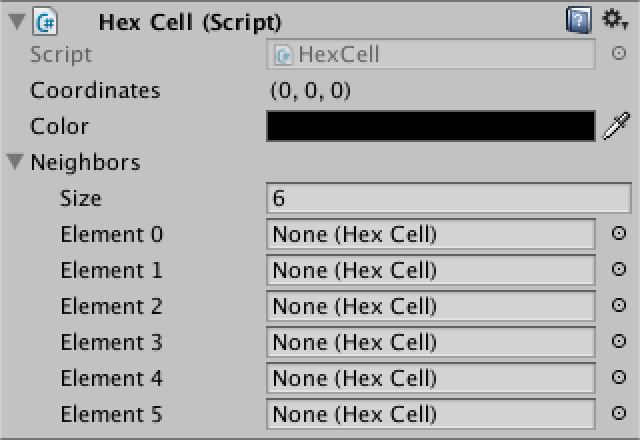Our prefab comes with room for six neighbors.

Now add a public method to retrieve a cell's neighbor in one direction. As a direction is always between 0 and 5, we don't need to check whether the index lies within the bounds of the array.

public HexCell GetNeighbor (HexDirection direction) {
return neighbors[(int)direction];
}

Add a method to set a neighbor too.

public void SetNeighbor (HexDirection direction, HexCell cell) {
neighbors[(int)direction] = cell;
}

Neighbor relationships are bidirectional. So when setting a neighbor in one direction, it makes sense to immediately set the neighbor in the opposite direction as well.

public void SetNeighbor (HexDirection direction, HexCell cell) {
neighbors[(int)direction] = cell;
cell.neighbors[(int)direction.Opposite()] = this;
}

Of course this assumes that we could ask a direction for its opposite. We can support this, by creating an extension method for HexDirection. To get the opposite direction, add 3 to the original direction. This only works for the first three directions though, for the others we have to subtract 3 instead.

public enum HexDirection {
NE, E, SE, SW, W, NW
}

public static class HexDirectionExtensions {

public static HexDirection Opposite (this HexDirection direction) {
return (int)direction < 3 ? (direction + 3) : (direction - 3);
}
}

Connecting Neighbors

We can initialize the neighbor relationship in HexGrid.CreateCell. As we go through the cells row by row, left to right, we know which cells have already been created. Those are the cells that we can connect to.

The simplest is the E–W connection. The first cell of each row doesn't have a west neighbor. But all other cells in the row do. And these neighbors have been created before the cell we're currently working with. So we can connect them.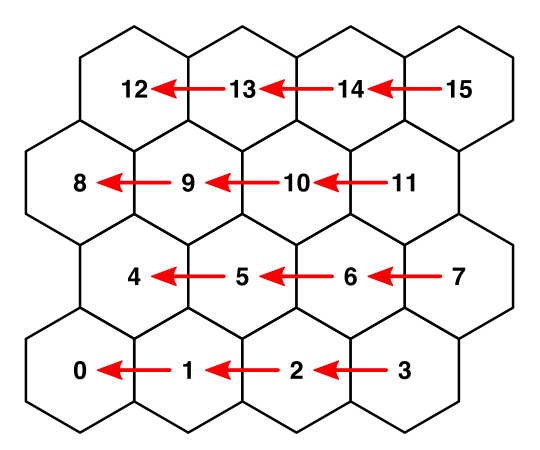Connecting from E to W as cells are created.
void CreateCell (int x, int z, int i) {
…
cell.color = defaultColor;

if (x > 0) {
cell.SetNeighbor(HexDirection.W, cells[i - 1]);
}

Text label = Instantiate<Text>(cellLabelPrefab);
…
}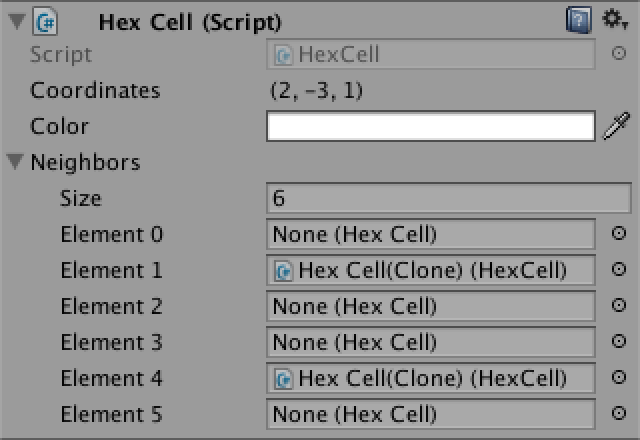East and west neighbors are connected.

We have two more bidirectional connections to make. As these are between different grid rows, we can only connect with the previous row. This means that we have to skip the first row entirely.

if (x > 0) {
cell.SetNeighbor(HexDirection.W, cells[i - 1]);
}
if (z > 0) {
}

As the rows zigzag, they have to be treated differently. Let's first deal with the even rows. As all cells in such rows have a SE neighbor, we can connect to those.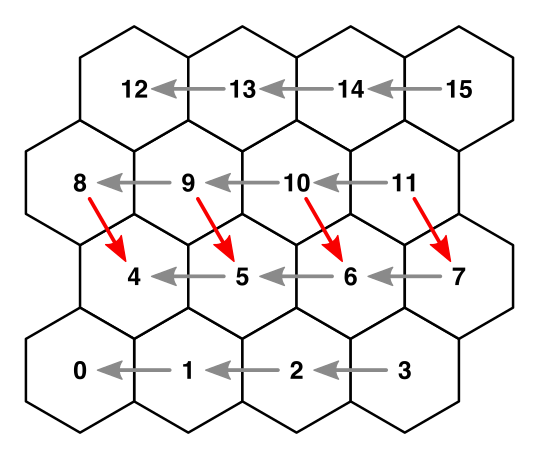Connecting from NW to SE on even rows.
if (z > 0) {
if ((z & 1) == 0) {
cell.SetNeighbor(HexDirection.SE, cells[i - width]);
}
}

We can connect to the SW neighbors as well. Except for the first cell in each row, as it doesn't have one.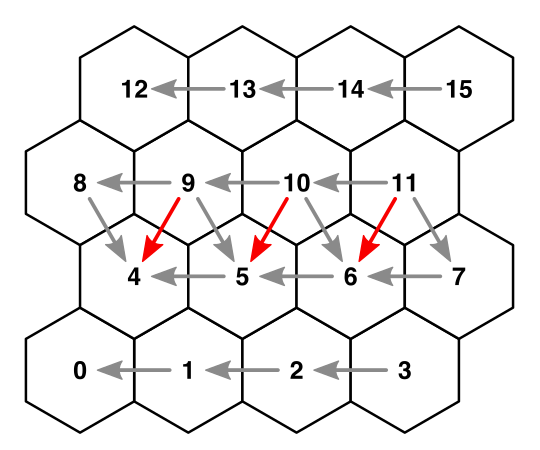Connecting from NE to SW on even rows.
if (z > 0) {
if ((z & 1) == 0) {
cell.SetNeighbor(HexDirection.SE, cells[i - width]);
if (x > 0) {
cell.SetNeighbor(HexDirection.SW, cells[i - width - 1]);
}
}
}

The odds rows follow the same logic, but mirrored. Once that's done, all neighbors in our grid are connected.

if (z > 0) {
if ((z & 1) == 0) {
cell.SetNeighbor(HexDirection.SE, cells[i - width]);
if (x > 0) {
cell.SetNeighbor(HexDirection.SW, cells[i - width - 1]);
}
}
else {
cell.SetNeighbor(HexDirection.SW, cells[i - width]);
if (x < width - 1) {
cell.SetNeighbor(HexDirection.SE, cells[i - width + 1]);
}
}
}

Of course not every cell is connected to exactly six neighbors. The cells that form the border of our grid end up with at least two and at most five neighbors. This is something that we have to be aware of.

unitypackage

Blending Colors

Color blending will make the triangulation of each cell more complex. So let's isolate the code of triangulating a single part. As we have directions now, let's use those to identify the parts, instead of a numeric index.

void Triangulate (HexCell cell) {
for (HexDirection d = HexDirection.NE; d <= HexDirection.NW; d++) {
Triangulate(d, cell);
}
}

void Triangulate (HexDirection direction, HexCell cell) {
Vector3 center = cell.transform.localPosition;
center,
center + HexMetrics.corners[(int)direction],
center + HexMetrics.corners[(int)direction + 1]
);
}

Now that we're using directions, it would be nice if we could fetch corners with directions, instead of having to convert to indices.

center,
center + HexMetrics.GetFirstCorner(direction),
center + HexMetrics.GetSecondCorner(direction)
);

This requires the addition of two static methods to HexMetrics. As a bonus, this allows us to make the corners array private.

static Vector3[] corners = {
new Vector3(0f, 0f, outerRadius),
new Vector3(0f, 0f, -outerRadius),
new Vector3(0f, 0f, outerRadius)
};

public static Vector3 GetFirstCorner (HexDirection direction) {
return corners[(int)direction];
}

public static Vector3 GetSecondCorner (HexDirection direction) {
return corners[(int)direction + 1];
}

Multiple Colors Per Triangle

Right now the HexMesh.AddTriangleColor method has a single color argument. This can only produce a triangle with a solid color. Let's add an alternative that supports a separate color for each vertex.

void AddTriangleColor (Color c1, Color c2, Color c3) {
}

Now we can start blending colors! Begin by simply using the neighbor's color for the other two vertices.

void Triangulate (HexDirection direction, HexCell cell) {
Vector3 center = cell.transform.localPosition;
center,
center + HexMetrics.GetFirstCorner(direction),
center + HexMetrics.GetSecondCorner(direction)
);
HexCell neighbor = cell.GetNeighbor(direction);
}

Unfortunately, this will produce a NullReferenceException because our border cells don't have six neighbors. What should we do when we lack a neighbor? Let's be pragmatic and use the cell itself as a substitute.

HexCell neighbor = cell.GetNeighbor(direction) ?? cell;

Color Averaging

Color blending works, but our current results are obviously incorrect. The color at the edges of our hexagons should be the average of the two adjacent cells.

HexCell neighbor = cell.GetNeighbor(direction) ?? cell;
Color edgeColor = (cell.color + neighbor.color) * 0.5f;

While we are now blending across edges, we still get sharp color boundaries. This happens because each vertex of a hexagon is shared by three hexagons in total.

This means that we also have to consider the neighbors of the previous and next directions. So we end up with four colors, in two sets of three.

Let's add two addition methods to HexDirectionExtensions to easily jump to the previous and next directions.

public static HexDirection Previous (this HexDirection direction) {
return direction == HexDirection.NE ? HexDirection.NW : (direction - 1);
}

public static HexDirection Next (this HexDirection direction) {
return direction == HexDirection.NW ? HexDirection.NE : (direction + 1);
}

Now we can retrieve all three neighbors and perform two three-way blends.

HexCell prevNeighbor = cell.GetNeighbor(direction.Previous()) ?? cell;
HexCell neighbor = cell.GetNeighbor(direction) ?? cell;
HexCell nextNeighbor = cell.GetNeighbor(direction.Next()) ?? cell;

cell.color,
(cell.color + prevNeighbor.color + neighbor.color) / 3f,
(cell.color + neighbor.color + nextNeighbor.color) / 3f
);

This produces correct color transitions, except along the border of the grid. The border cells don't agree on the colors of the missing neighbors, so you still see sharp boundaries there. Overall though, our current approach doesn't produce a pleasing result. We need a better strategy.

unitypackage

Blend Regions

Blending across the entire surface of a hexagon leads to a blurry mess. You can no longer clearly see the individual cells. We can improve this a lot by only blending near the edges of hexagons. That leaves an inner hexagonal region with a solid color.

How large should this solid region be, versus the blend region? Different distributions lead to different visuals. We can define this region as a fraction of the outer radius. Let's make it 75%. This leads to two new metrics, which add up to 100%.

public const float solidFactor = 0.75f;

public const float blendFactor = 1f - solidFactor;

With the new solid factor, we can create methods to retrieve the corners of solid inner hexagons.

public static Vector3 GetFirstSolidCorner (HexDirection direction) {
return corners[(int)direction] * solidFactor;
}

public static Vector3 GetSecondSolidCorner (HexDirection direction) {
return corners[(int)direction + 1] * solidFactor;
}

Now change HexMesh.Triangulate so it uses these solid corners instead of the original ones. Leave the colors as they are for now.

center,
center + HexMetrics.GetFirstSolidCorner(direction),
center + HexMetrics.GetSecondSolidCorner(direction)
);

Triangulating Blend Regions

We need to fill the empty space that we have created by shrinking our triangles. This space has the shape of a trapezoid in each direction. We can use a quad to cover it. So create methods to add a quad and its colors.

void AddQuad (Vector3 v1, Vector3 v2, Vector3 v3, Vector3 v4) {
int vertexIndex = vertices.Count;
}

void AddQuadColor (Color c1, Color c2, Color c3, Color c4) {
}

Rework HexMesh.Triangulate so the triangle gets a single color and the quad blends between the solid color and the two corner colors.

void Triangulate (HexDirection direction, HexCell cell) {
Vector3 center = cell.transform.localPosition;
Vector3 v1 = center + HexMetrics.GetFirstSolidCorner(direction);
Vector3 v2 = center + HexMetrics.GetSecondSolidCorner(direction);

Vector3 v3 = center + HexMetrics.GetFirstCorner(direction);
Vector3 v4 = center + HexMetrics.GetSecondCorner(direction);

HexCell prevNeighbor = cell.GetNeighbor(direction.Previous()) ?? cell;
HexCell neighbor = cell.GetNeighbor(direction) ?? cell;
HexCell nextNeighbor = cell.GetNeighbor(direction.Next()) ?? cell;

cell.color,
cell.color,
(cell.color + prevNeighbor.color + neighbor.color) / 3f,
(cell.color + neighbor.color + nextNeighbor.color) / 3f
);
}

Edge Bridges

This is starting to look better, but we're still not there yet. The color blend between two neighbors gets polluted by the cells adjacent to the edge. To prevent this, we have to cut the corners from our trapezoid and turn it into a rectangle. It then forms a bridge between a cell and its neighbor, leaving gaps at the sides.

We can find the new positions of v3 and v4 by starting from v1 and v2, then moving along the bridge, straight to the edge of the cell. So what is this bridge offset? You can find it by taking the midpoint between the two relevant corners, then applying the blend factor to that. This is a job for HexMetrics.

public static Vector3 GetBridge (HexDirection direction) {
return (corners[(int)direction] + corners[(int)direction + 1]) *
0.5f * blendFactor;
}

Back to HexMesh, it now makes sense to add a variant of AddQuadColor that only needs two colors.

void AddQuadColor (Color c1, Color c2) {
}

Adjust Triangulate so it creates correctly blending bridges between neighbors.

Vector3 bridge = HexMetrics.GetBridge(direction);
Vector3 v3 = v1 + bridge;
Vector3 v4 = v2 + bridge;

HexCell prevNeighbor = cell.GetNeighbor(direction.Previous()) ?? cell;
HexCell neighbor = cell.GetNeighbor(direction) ?? cell;
HexCell nextNeighbor = cell.GetNeighbor(direction.Next()) ?? cell;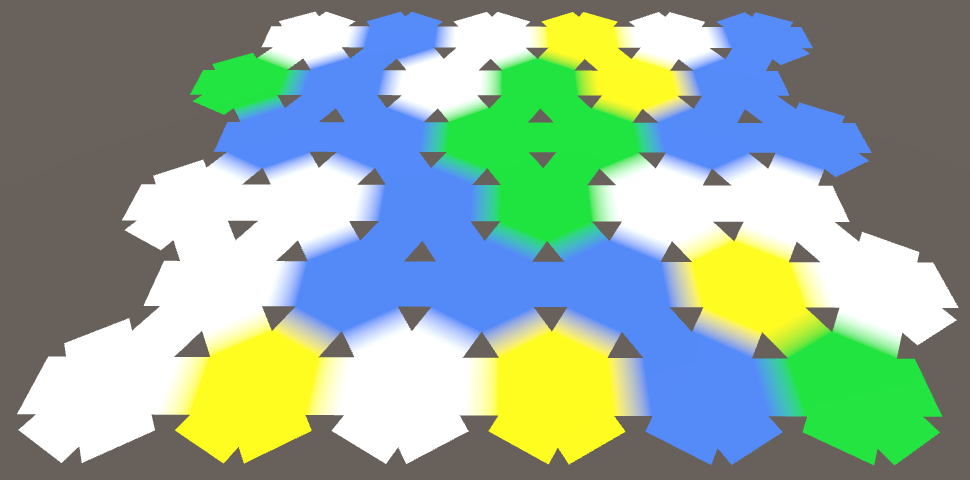Correctly colored bridges, with corner gaps.

Filling the Gaps

We are now left with a triangular gap wherever three cells meet. We got those holes by cutting off the triangular sides of our trapezoids. So let's add those triangles back.

First consider the triangle that connects with the previous neighbor. Its first vertex has the cell's color. Its second vertex's color is a three-color blend. And the final vertex has the same color as halfway across the bridge.

Color bridgeColor = (cell.color + neighbor.color) * 0.5f;

AddTriangle(v1, center + HexMetrics.GetFirstCorner(direction), v3);
cell.color,
(cell.color + prevNeighbor.color + neighbor.color) / 3f,
bridgeColor
);

Finally, the other triangle work the same way, except that its second vertex touches the bridge, instead of its third vertex.

AddTriangle(v2, v4, center + HexMetrics.GetSecondCorner(direction));
cell.color,
bridgeColor,
(cell.color + neighbor.color + nextNeighbor.color) / 3f
);

Now we have nice blend regions that we can give any size that we want. Fuzzy or crisp cell edges, it is up to you. But you'll notice that the blending near the grid border is still not correct. Again, we will let that rest for now, and instead focus our attention on another matter.

unitypackage

Fusing Edges

Take a look at the topology of our grid. What distinct shapes are there? If we ignore the border, then we can identify three distinct shape types. There are single-color hexagons, dual-color rectangles, and triple-color triangles. You find all three shapes wherever three cells meet.

So every two hexagons are connected by a single rectangular bridge. And every three hexagons are connected by a single triangle. However, we triangulate in a more complex way. We currently use two quads to connect a pair of hexagons, instead of just one. And we're using a total of six triangles to connect three hexagons. That seems excessive. Also, if we were to directly connect with a single shape, we wouldn't need to do any color averaging. So we could get away with less complexity, less work, and less triangles.

Direct Bridges

Our edge bridges right now consist of two quads. To make them go all the way across to the next hexagon, we have to double the bridge length. This means that we no longer have to average the two corners in HexMetrics.GetBridge. Instead we just add them and then multiply with the blend factor.

public static Vector3 GetBridge (HexDirection direction) {
return (corners[(int)direction] + corners[(int)direction + 1]) *
blendFactor;
}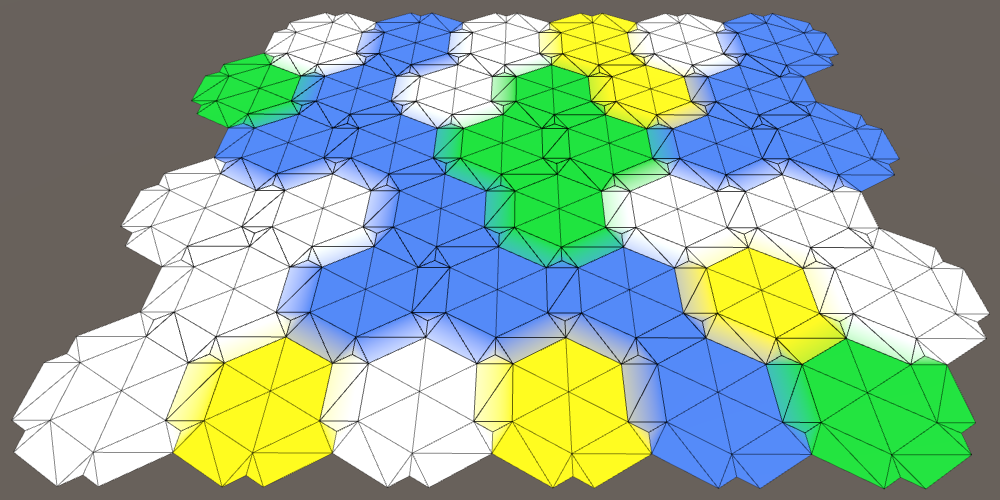Bridges go all the way across, and overlap.

The bridges now form direct connections between hexagons. But we're still generating two per connection, one in each direction, which overlap. So only one of the two cells has to create a bridge between them.

Let's first simplify our triangulation code. Remove everything that deals with the edge triangles and color blending. Then move the code that adds the bridge quad to a new method. Pass the first two vertices to this method, so we don't have to derive them again.

void Triangulate (HexDirection direction, HexCell cell) {
Vector3 center = cell.transform.localPosition;
Vector3 v1 = center + HexMetrics.GetFirstSolidCorner(direction);
Vector3 v2 = center + HexMetrics.GetSecondSolidCorner(direction);

TriangulateConnection(direction, cell, v1, v2);
}

void TriangulateConnection (
HexDirection direction, HexCell cell, Vector3 v1, Vector3 v2
) {
HexCell neighbor = cell.GetNeighbor(direction) ?? cell;

Vector3 bridge = HexMetrics.GetBridge(direction);
Vector3 v3 = v1 + bridge;
Vector3 v4 = v2 + bridge;

}

Now we can easily limit the triangulation of connections. Start by only adding a bridge when we're dealing with a NE connection.

if (direction == HexDirection.NE) {
TriangulateConnection(direction, cell, v1, v2);
}

It looks like we can cover all connections by only triangulating them in the first three directions. So NE, E, and SE.

if (direction <= HexDirection.SE) {
TriangulateConnection(direction, cell, v1, v2);
}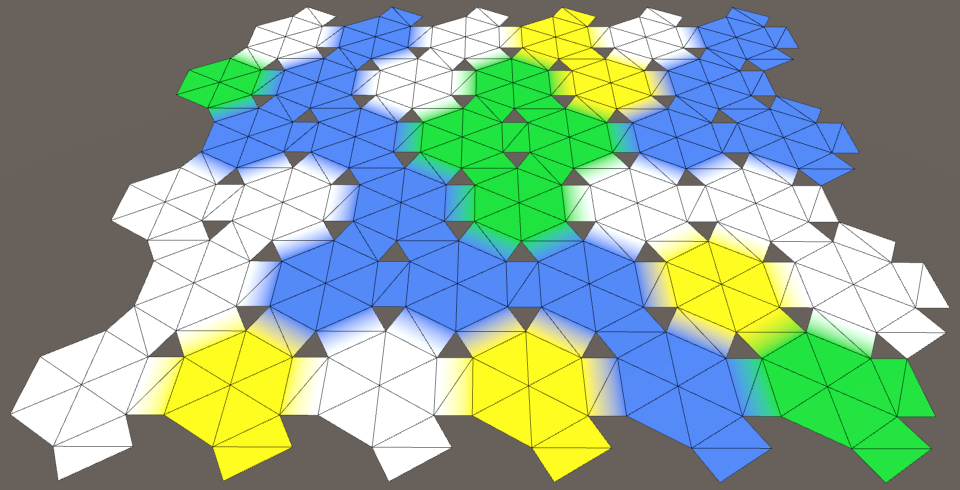All internal bridges, some border bridges.

All connections between two adjacent cells are now covered. But we also got some bridges that lead out of the grid, to nowhere. Let's get rid of those, by bailing out of TriangulateConnection when we end up with no neighbor. So we no longer replace missing neighbors with the cell itself.

void TriangulateConnection (
HexDirection direction, HexCell cell, Vector3 v1, Vector3 v2
) {
HexCell neighbor = cell.GetNeighbor(direction);
if (neighbor == null) {
return;
}

…
}

Triangular Connections

We have to plug the triangular holes again. Let's do this for the triangle that connects to the next neighbor. Once again, we should only do this if that neighbor actually exists.

void TriangulateConnection (
HexDirection direction, HexCell cell, Vector3 v1, Vector3 v2
) {
…

HexCell nextNeighbor = cell.GetNeighbor(direction.Next());
if (nextNeighbor != null) {
}
}

What is the position of the third vertex? I put in v2 as a placeholder, but that is obviously not correct. As each edge of these triangles connects with a bridge, we can find it by traveling along the bridge of the next neighbor.

AddTriangle(v2, v4, v2 + HexMetrics.GetBridge(direction.Next()));